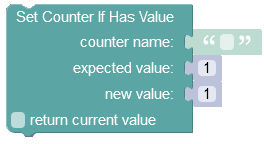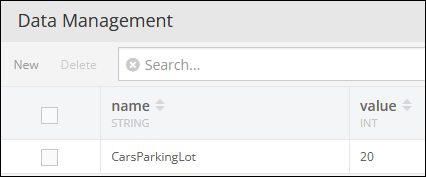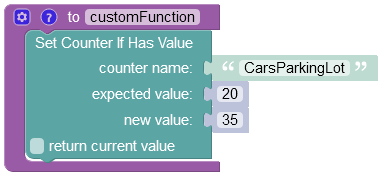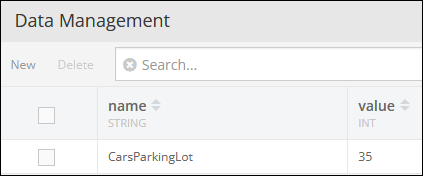# Conditional update¶

Atomically sets the value to the given updated value if the current value == the expected value.

``````Future<bool> Backendless.counters.compareAndSet(String counterName, int expected, int updated);

IAtomic counter = Backendless.counters.of(String counterName);
Future<bool> counter.compareAndSet(int expected, int updated);
``````

where:

Argument                Description
`counterName` name of the counter to compare.
`expected` the expected value of the counter. If the current value equals the expected value, the counter is set to the "`updated`" value.
`updated` the new value to assign to the counter if the current value equals the `expected` value.

#### Example¶

``````Function callback = (result) => print("value has been updated - \$result");

Backendless.counters.compareAndSet("my counter", 1000, 2000).then(callback);

IAtomic myCounter = Backendless.counters.of("my counter");
myCounter.compareAndSet(1000, 2000).then(callback);
``````

### Codeless Reference¶where:

Argument                Description
`counter name` Name of the counter whose value must be set.
`expected value` This property sets the following condition: If the expected value equals to the current value of the counter, then the operation sets a new number for the counter. Otherwise, the operation does not set the new value for the counter.
`new value` Specify the new number for the counter.
`return current value` When this box is checked, the operation returns the current value of the counter.

Returns the current value of the counter.

Consider the following counter:The example below checks if the expected value of the `"CarsParkingLot"` counter equals to `20`, and if it does equal to `20`, then the operation sets a new value to `35`.The result of this operation will look as shown below after the Codeless logic runs: